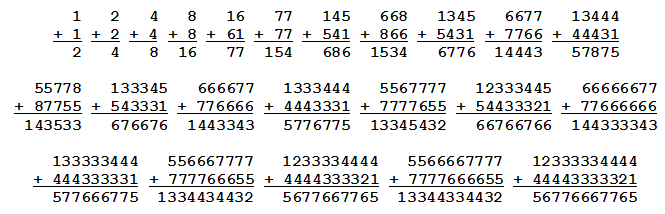# Conway’s RATS Sequence

Write down an integer. Remove any zeros and sort the digits in increasing order. Now add this number to its reversal to produce a new number, and perform the same operations on that:In the example above, we’ve arrived at a pattern, alternating between 12333334444 and 5566667777 but adding a 3 and a 6 (respectively) with each iteration. (So the next two sums, after their digits are sorted, will be 123333334444 and 55666667777, and so on.)

Princeton mathematician John Horton Conway calls this the RATS sequence (for “reverse, add, then sort”) and in 1989 conjectured that no matter what number you start with (in base 10), you’ll either enter the divergent pattern above or find yourself in some cycle. For example, starting with 3 gives:

3, 6, 12, 33, 66, 123, 444, 888, 1677, 3489, 12333, 44556, 111, 222, 444, …

… and now we’re in a loop — the last eight terms will just repeat forever.

Conway’s colleague at Princeton, Curt McMullen, showed that the conjecture is true for all numbers less than a hundred million, and himself conjectured that every RATS sequence in bases smaller than 10 is eventually periodic. Are they right? So far neither conjecture has been disproved.

(Richard K. Guy, “Conway’s RATS and Other Reversals,” American Mathematical Monthly 96:5 [May 1989], 425-428.)